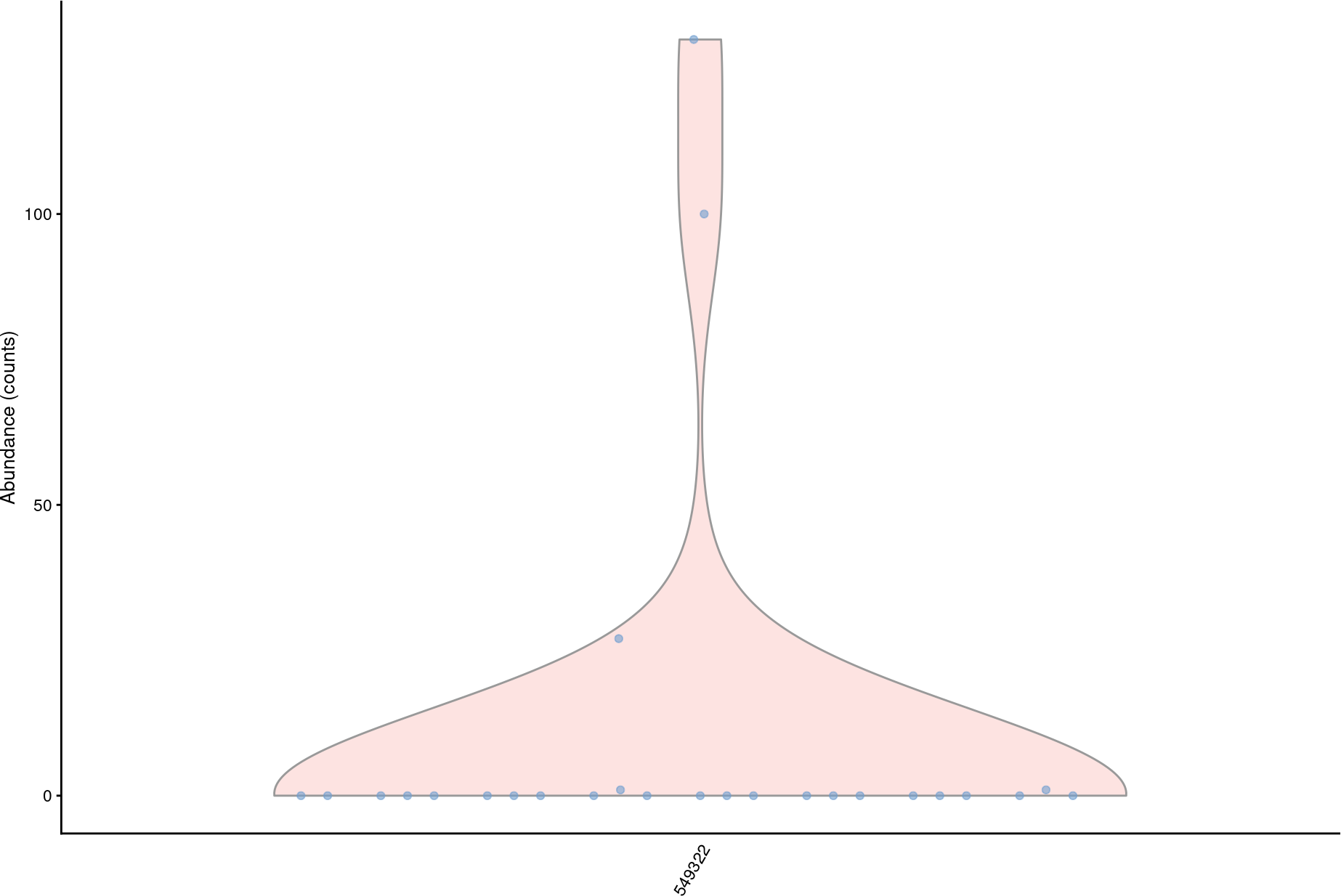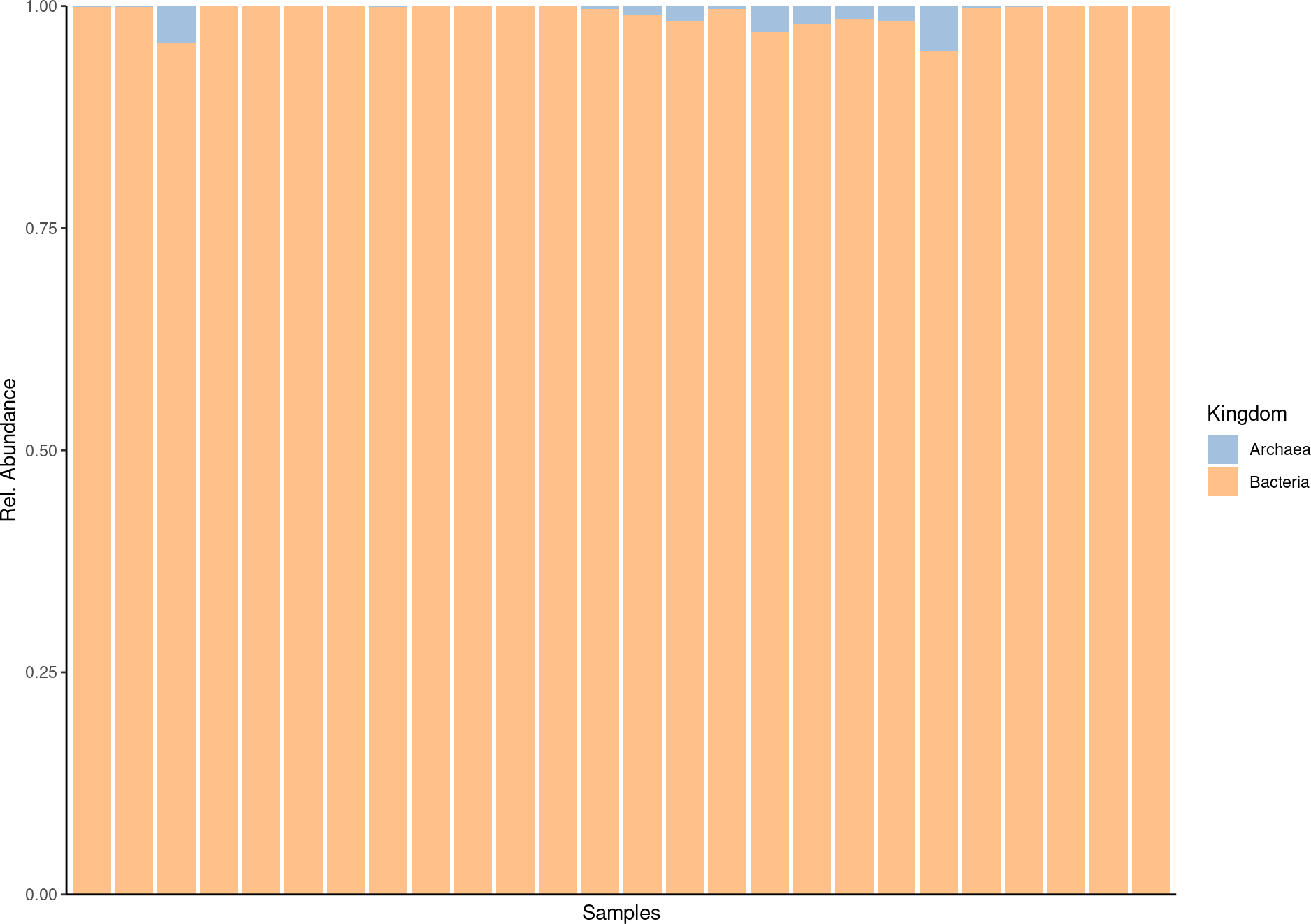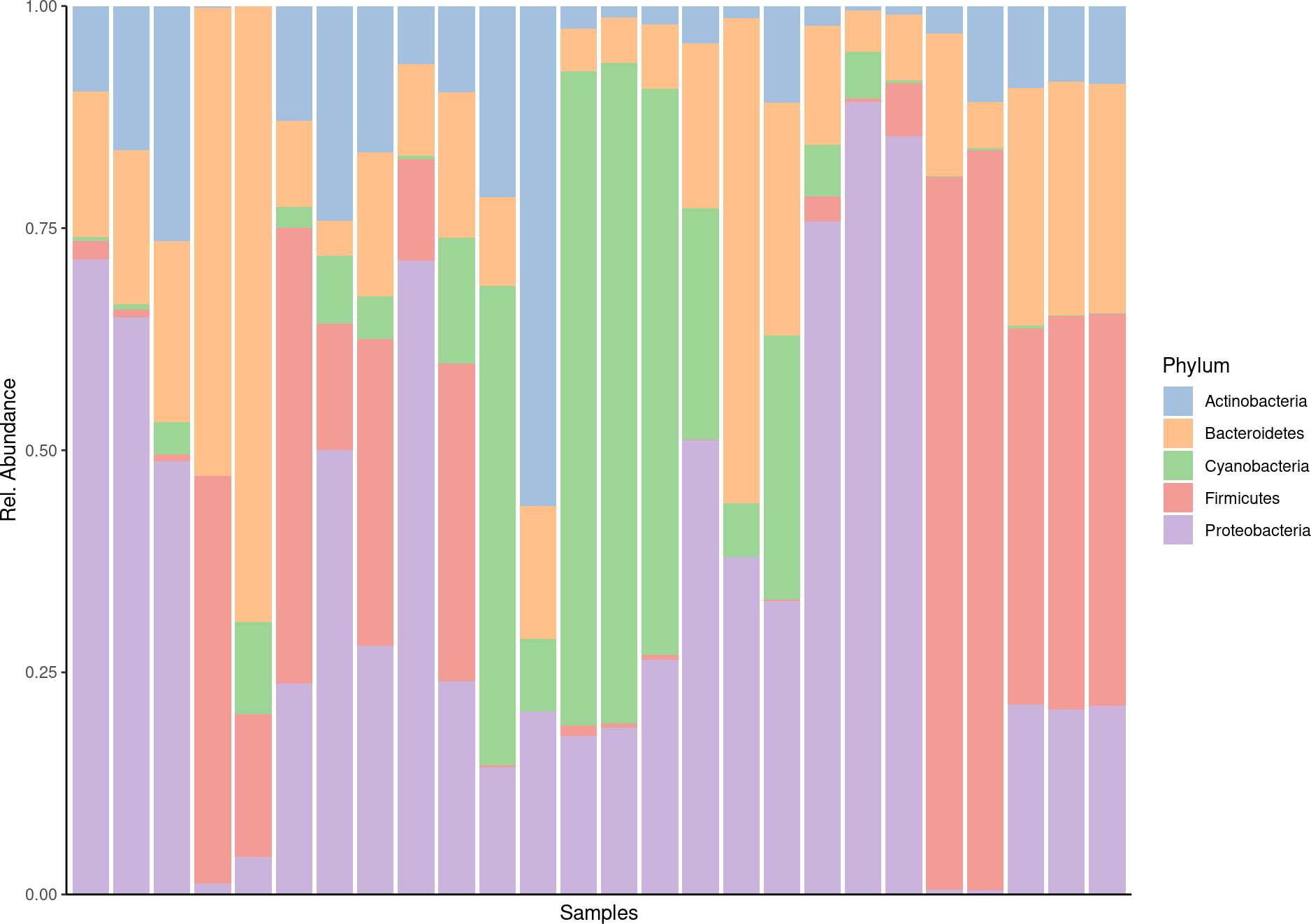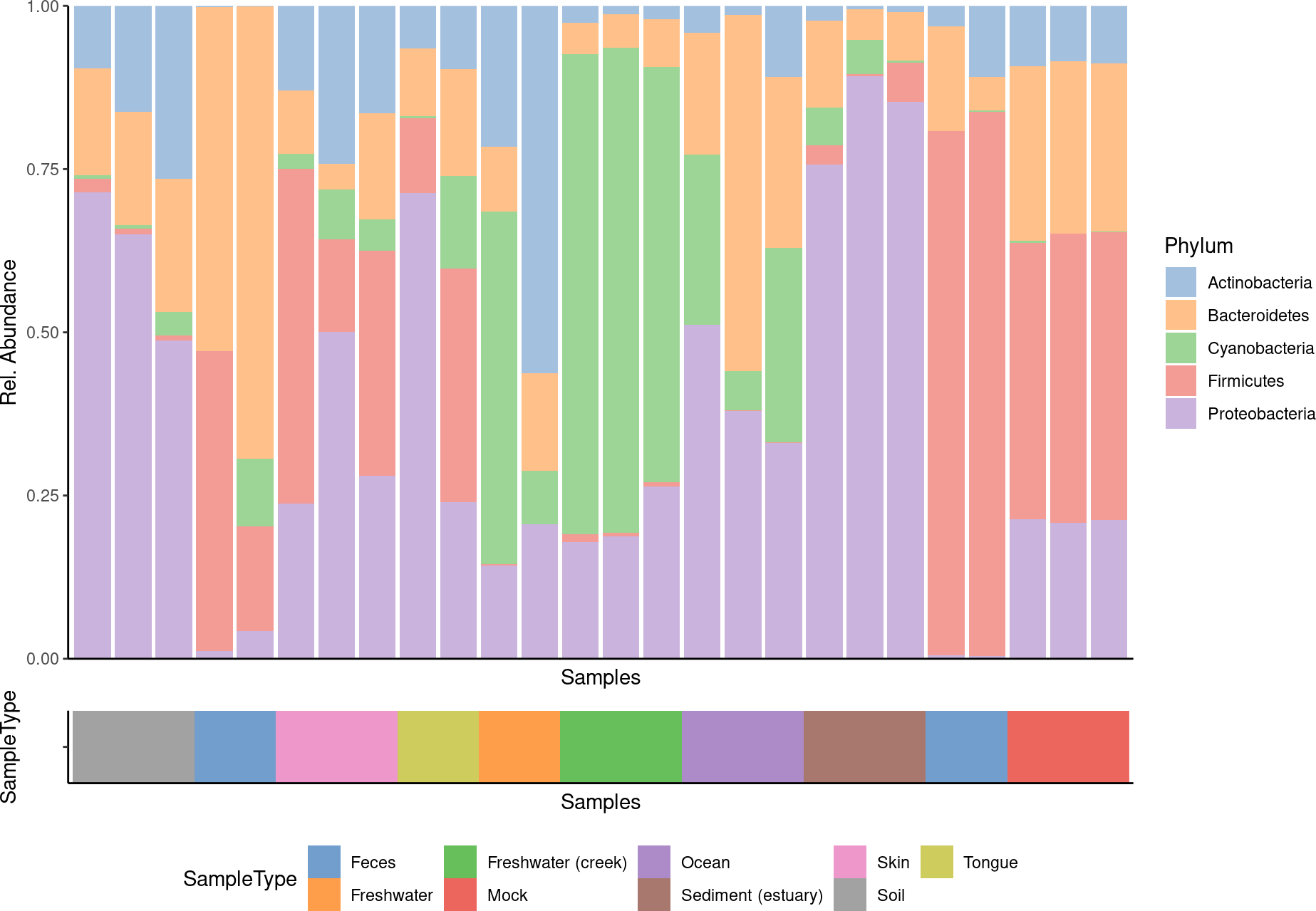# Contents

`miaViz` implements plotting function to work with `TreeSummarizedExperiment` and related objects in a context of microbiome analysis. For more general plotting function on `SummarizedExperiment` objects the `scater` package offers several options, such as `plotColData`, `plotExpression` and `plotRowData`.

# 1 Installation

To install `miaViz`, install `BiocManager` first, if it is not installed. Afterwards use the `install` function from `BiocManager` and load `miaViz`.

``````if (!requireNamespace("BiocManager", quietly = TRUE))
install.packages("BiocManager")

BiocManager::install("miaViz")
#> Bioconductor version 3.13 (BiocManager 1.30.16), R 4.1.0 (2021-05-18)
#> Warning: package(s) not installed when version(s) same as current; use `force = TRUE` to
#>   re-install: 'miaViz'``````
``````library(miaViz)
data(GlobalPatterns, package = "mia")``````

# 2 Abundance plotting

in contrast to other fields of sequencing based fields of research for which expression of genes is usually studied, microbiome research uses the more term Abundance to described the numeric data measured and analyzed. Technically, especially in context of `SummarizedExperiment` objects, there is no difference. Therefore `plotExpression` can be used to plot `Abundance` data.

`plotAbundance` can be used as well and as long as `rank` is set `NULL`, it behaves as `plotExpression`.

``````plotAbundance(GlobalPatterns, rank = NULL,
features = "549322", abund_values = "counts")``````However, if the `rank` is set not `NULL` a bar plot is returned. At the same time the `features` argument can be set to `NULL` (default).

``GlobalPatterns <- transformCounts(GlobalPatterns, method = "relabundance")``
``plotAbundance(GlobalPatterns, rank = "Kingdom", abund_values = "relabundance")``With subsetting to selected features the plot can be fine tuned.

``````prev_phylum <- getPrevalentTaxa(GlobalPatterns, rank = "Phylum",
detection = 0.01)``````
``````plotAbundance(GlobalPatterns[rowData(GlobalPatterns)\$Phylum %in% prev_phylum],
rank = "Phylum",
abund_values = "relabundance")``````The `features` argument is reused for plotting data along the different samples. In the next example the SampleType is plotted along the samples. In this case the result is a list, which can combined using external tools, for example `patchwork`.

``````library(patchwork)
plots <- plotAbundance(GlobalPatterns[rowData(GlobalPatterns)\$Phylum %in% prev_phylum],
features = "SampleType",
rank = "Phylum",
abund_values = "relabundance")
plots\$abundance / plots\$SampleType +
plot_layout(heights = c(9, 1))``````# 3 Prevalence plotting

To visualize prevalence within the dataset, two functions are available, `plotTaxaPrevalence`, `plotPrevalenceAbundance` and `plotPrevalence`.

`plotTaxaPrevalence` produces a so-called landscape plot, which visualizes the prevalence of samples across abundance thresholds.

``````plotTaxaPrevalence(GlobalPatterns, rank = "Phylum",
detections = c(0, 0.001, 0.01, 0.1, 0.2))``````